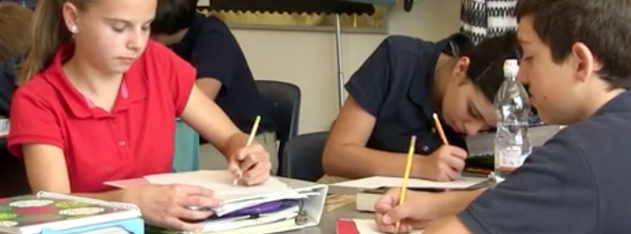# Math

•St. Agatha Catholic School middle school is designed to prepare students for what lays ahead … high school! Each grade has a homeroom where they go in the morning, get settled and start their day with prayer.  From there they move from class to class, subject to subject. With five basic subjects and four specialty subjects and an elective, there are seven periods each day.

•• Key Areas of Study

• Develop fluency with multiplication and division of fractions and decimals
• Apply the order of operations and properties of addition and multiplication
• Solve problems with ratio, rate and percent
• Understand probability and represent it with ratios, decimals, and percents
• Develop meanings and uses of variables
• Use expressions and formulas
• Represent relationships and patterns with tables, graphs, and words
• Solve one-step equations

• Compute with integers and rational numbers
• Solve linear equations
• Represent proportional relationships with graphs and tables.
• Apply ratios and proportions to solve percent and probability problems
• Convert different units of measurement to solve rate problems
• Use formulas to determine surface area and volume
• Solve one-step equations

• Analyze and represent linear functions in algebraic and graph forms
• Understand and determine slope of a line
• Relate systems of two linear equations to pairs of lines
• Organize and display data to answer questions
• Analyze data displays and descriptive statistics
• Use and analyze statistical measures for appropriateness
• Analyze two and three dimensional spaces and figures
• Use the Triangle Sum Theorem and the Pythagorean Theorem